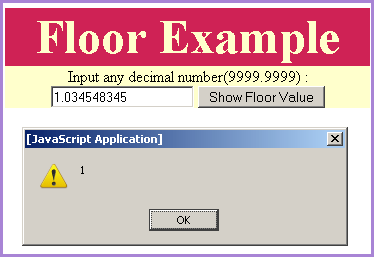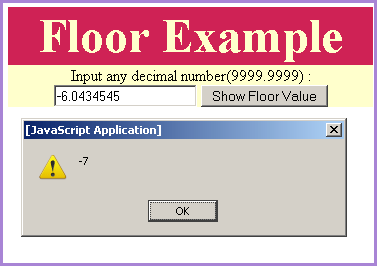Tutorials

JavaScript floor method

JavaScript floor method is used to achieve the next complete number in the downward position.

JavaScript floor method is used to achieve the next complete number in the downward position.

JavaScript floor method

JavaScript floor method is used to achieve the next complete number in the downward position. For example if we apply the floor method on the number like "1.2213" then it will return the floor value as "1" and if we will provide some negative number as "-4.463465" then it will return the number as "-5".

Syntax:

 Math.floor( number) ;

Description of code:

We have created an input text box in which user can provide the number of which we have to calculate the floor value. When user clicks on the button "Show Floor value" it calls the method callFloor() . In the callFloor() method it takes the number from the text box and shows the floor value in the alert message by using the alert() method. Here is the full example code of floorExample.html as follows:


Floor Example
Input any decimal number(9999.9999) :

Output :Input any number to find its floor value.